## 13) Missing Number Equations

Bonds of 5: Fill a Row### Mathematics

Develop fluency using two-part bonds of 5 in related addition or subtraction equation where the unknown is a missing part.

### Language

• addition as ‘and’. Read aloud as “(Part) and (part) is 5”.
• subtraction as ‘take away’. Read aloud as “(Whole) take away (part) is (part)”.
• equals as ‘is’
• subtraction solved using adding on. For example, 5 – ? = 3 can be thought of as “What part joins (adds on) to 3 to make the whole of 5?”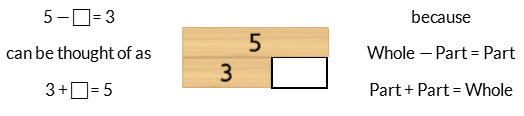## Differentiation

### A little easier

##### One column play

Play using the first column on each player’s game board. The player who fills the most missing numbers in 3 minutes wins. This is helpful for students who need to focus on repeating the same type of question to develop fluency.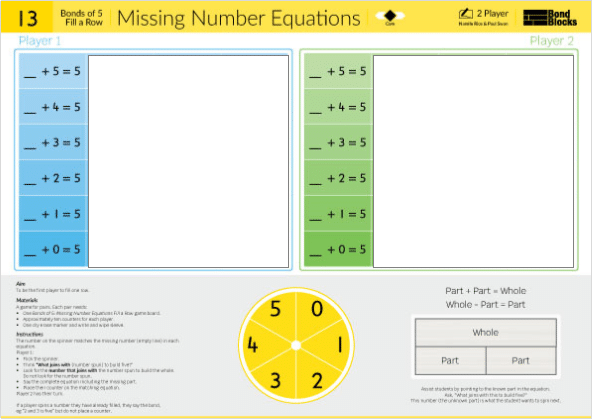Repeat using different columns. Each column has the missing number in a different position.##### Build a wall
• Cover the bottom three rows with a piece of paper.
• Build a two-part bond wall of 5. Use one of each block from 1 to 5. Place this next to each related row on the activity board.
• Use the blocks to solve each missing number in the first row. Write each missing number on the write and wipe sleeve with a dry erase marker.
• Repeat for row 2, then row 3.
• Discuss patterns. For example, each row uses the same two-part bond of 5.
• Predict what missing number equations will be on the forth row. (Note vertical counting patterns.) Write these equations on the sleeve. Then move the covering piece of paper and check.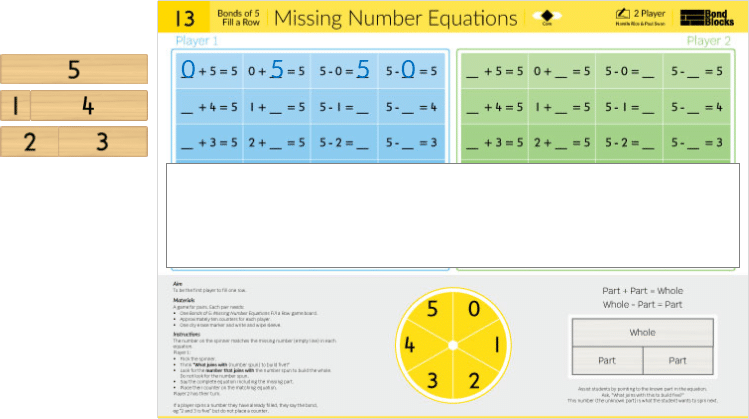##### Three row play

Playing using the first three rows. Cover the bottom three rows. This is helpful for students who are visually overwhelmed by the number of playing spaces to scan.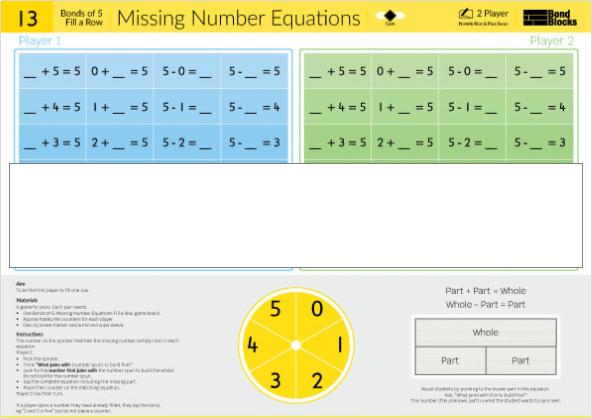Repeat using the bottom three rows. Cover the top three rows.

##### Card sort

Click to download the Bonds of 5 Missing Number Equations Card Sort. Each card is one of the equations from the Bonds of 5 Missing Number Equations game board. Instruct students to:

• Build a wall of 5 with Bond Blocks and use this to fill in the missing number boxes.
• Cut out each card. Sort the cards into groups that are made up of the same two-part bonds, using the part-part-whole diagram heading cards.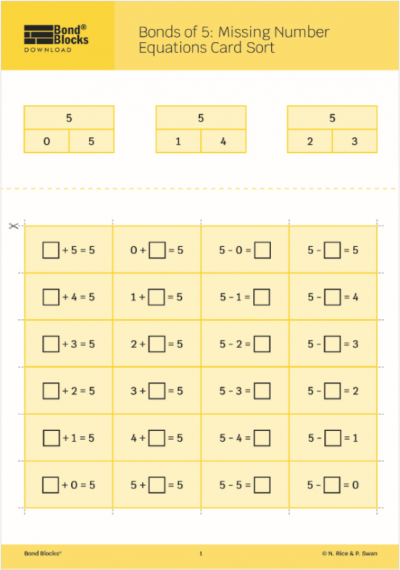### Progression

In the next activity students apply the skills of representing of Bonds of 5 with Bond Blocks and as addition and subtraction equations to create related number stories using words and pictures. Go to

##### Activity 14

Bonds of 5: Representing Addition, Thinkboard

Bonds of 5: Representing Subtraction, Thinkboard